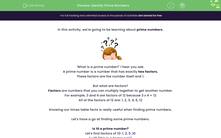# Identify Prime Numbers

In this worksheet, students will learn how to identify prime numbers.Key stage:  KS 2

Curriculum topic:   Number: Addition, Subtraction, Multiplication and Division

Curriculum subtopic:   Identify Common Factors, Multiples and Prime Numbers

Difficulty level:#### Worksheet Overview

In this activity, we're going to be learning about prime numbers.'What is a prime number?' I hear you ask.

A prime number is a number that has exactly two factors.

These factors are the number itself and 1.

But what are factors?

Factors are numbers that you can multiply together to get another number.

For example, 3 and 4 are factors of 12 because 3 x 4 = 12

All of the factors of 12 are: 1, 2, 3, 4, 6, 12

Knowing our times table facts is really useful when finding prime numbers.

Let's have a go at finding some prime numbers.

Is 10 a prime number?

Let's find factors of 10: 1, 2, 5 ,10

1 x 10 (this is a factor pair)

2 x 5  (this is a factor pair)

We can see that 10 has four factors so is not a prime number.

Is 7 a prime number?

Let's find the factors of 7:  1, 7

1 x 7 (this is a factor pair)

The only factors of 7 are 7 itself and 1.

7 is a prime number.

Now why don't you have a go at finding some prime numbers?

Remember:

Factors are numbers that you can multiply together to get another number.

A prime number is a number that has only two factors:  itself and 1.

Come back and have a look at this page if you get stuck! Just click on the red help button on the screen.Good luck!

### What is EdPlace?

We're your National Curriculum aligned online education content provider helping each child succeed in English, maths and science from year 1 to GCSE. With an EdPlace account you’ll be able to track and measure progress, helping each child achieve their best. We build confidence and attainment by personalising each child’s learning at a level that suits them.

Get started